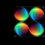# Remainder???

Hey Brilliantians!!

I just wanted to know how do you solve problems related to finding remainders? Like example- Find the remainder when 1000! is divided by 1000. Please can anyone tell me how to do these kind of problemsNote by Anuj Shikarkhane
6 years, 11 months ago

This discussion board is a place to discuss our Daily Challenges and the math and science related to those challenges. Explanations are more than just a solution — they should explain the steps and thinking strategies that you used to obtain the solution. Comments should further the discussion of math and science.

When posting on Brilliant:

• Use the emojis to react to an explanation, whether you're congratulating a job well done , or just really confused .
• Ask specific questions about the challenge or the steps in somebody's explanation. Well-posed questions can add a lot to the discussion, but posting "I don't understand!" doesn't help anyone.
• Try to contribute something new to the discussion, whether it is an extension, generalization or other idea related to the challenge.

MarkdownAppears as
*italics* or _italics_ italics
**bold** or __bold__ bold
- bulleted- list
• bulleted
• list
1. numbered2. list
1. numbered
2. list
Note: you must add a full line of space before and after lists for them to show up correctly
paragraph 1paragraph 2

paragraph 1

paragraph 2

[example link](https://brilliant.org)example link
> This is a quote
This is a quote
    # I indented these lines
# 4 spaces, and now they show
# up as a code block.

print "hello world"
# I indented these lines
# 4 spaces, and now they show
# up as a code block.

print "hello world"
MathAppears as
Remember to wrap math in $$ ... $$ or $ ... $ to ensure proper formatting.
2 \times 3 $2 \times 3$
2^{34} $2^{34}$
a_{i-1} $a_{i-1}$
\frac{2}{3} $\frac{2}{3}$
\sqrt{2} $\sqrt{2}$
\sum_{i=1}^3 $\sum_{i=1}^3$
\sin \theta $\sin \theta$
\boxed{123} $\boxed{123}$

Sort by:

- 6 years, 11 months ago

My also comment got downvoted.

- 6 years, 11 months ago

See this post. Read about Euler's Theorem and its special case called Fermat's Little Theorem. Also read about Wilson's theorem, Chinese Remainder Theorem (Extended Euclidean Algorithm is related to it, read about it too.). They are all very useful and worth learning if you want to master modular arithmetic.

The less useful one is the Euler's Theorem's equivalent but with the Carmichael's function $\lambda$ used instead of the Euler's Totient function $\phi$. We have $\lambda\le \phi$, which means the Carmichael's function is 'stronger' sometimes - we could occasionally reduce the exponent more than using the Euler's totient function. You can read more about it and a lot of other stuff here. It is not a popular theorem, though.

- 6 years, 11 months ago

Thanks I would do it.It's a little tough but I can manage

- 6 years, 11 months ago

@Calvin Lin I can't see why my comment got downvoted. If you have an idea why, it'd be great to know.

- 6 years, 11 months ago

Hey there...1000! is divisible by 1000...so remainder is zero...but for more complex problems, u should look up the results and theoroms related to modular mathematics..it should help..

- 6 years, 11 months ago

How can you say that 1000! is divisible by 1000??

- 6 years, 11 months ago

$1000!=1000\times 999\times 998\times \cdots\times 2\times 1$ by the definition of a factorial.

- 6 years, 11 months ago